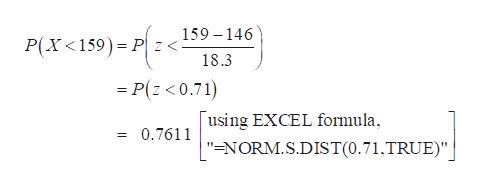# Suppose that the number of Facebook friends users have is normally distributed with a mean of 146 and a standard deviation of about 58. Assume ten individuals are randomly chosen. Answer the following questions. Round all answers to 4 decimal places where possible.For part b), is the assumption that the distribution is normal necessary? YesNo

Question
1 views

Suppose that the number of Facebook friends users have is normally distributed with a mean of 146 and a standard deviation of about 58. Assume ten individuals are randomly chosen. Answer the following questions. Round all answers to 4 decimal places where possible.

For part b), is the assumption that the distribution is normal necessary? YesNo

check_circle

Step 1

Standard normal distribution:

The standard normal distribution is a special case of normal distribution, in which the mean of the distribution is 0 and standard deviation of the distribution is 1. That is X~N(µ, σ). The Z-scores can be calculated using the given formula.help_outlineImage Transcriptionclosewhere, x is the normal random variable is the mean, and o is the standard deviation fullscreen
Step 2

In this context, it is given that the mean number of Facebook friend’s users is 146 corresponding to its standard deviation of 58.

Denote X as the number of Facebook friends users.

In general, X follows normal with mean value of 146 and standard deviation of 58. That is X~...help_outlineImage Transcriptionclose159 146 P(X 159) P Z< 18.3 =P(z<0.71) | using EXCEL formula 0.7611 = "ENORM.S.DIST(0.71,TRUE)" fullscreen

### Want to see the full answer?

See Solution

#### Want to see this answer and more?

Solutions are written by subject experts who are available 24/7. Questions are typically answered within 1 hour.*

See Solution
*Response times may vary by subject and question.
Tagged in

### Hypothesis Testing